Courses

# Examples : Euler-Lagrange Equation (Part - 2) - Classical Mechanics, CSIR-NET Physical Sciences Physics Notes | EduRev

## Physics for IIT JAM, UGC - NET, CSIR NET

Created by: Akhilesh Thakur

## Physics : Examples : Euler-Lagrange Equation (Part - 2) - Classical Mechanics, CSIR-NET Physical Sciences Physics Notes | EduRev

The document Examples : Euler-Lagrange Equation (Part - 2) - Classical Mechanics, CSIR-NET Physical Sciences Physics Notes | EduRev is a part of the Physics Course Physics for IIT JAM, UGC - NET, CSIR NET.
All you need of Physics at this link: Physics

Example 8 : Show that the time taken by a particle moving along a curve y = y ( x )
with velocity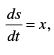om the point (0,0) to the point (1,1) is minimum if the curve is a circle having its center on the x-axis.

Solution: Let a particle be moving along a curve y = y(x) from the point (0, 0) to the point  (1, 1) with velocity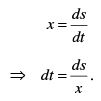Therefore the total time required for the particle to move from the point (0, 0) to the point (1, 1) is given by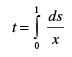. . . (1)
where the infinitesimal distance between two points on the path is given by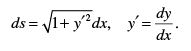Hence the equation (1) becomes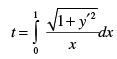. . . (2)

Time t is minimum if the integrand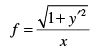. . . (3)
must satisfy the Euler-Lagrange’s equation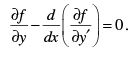. . . (4)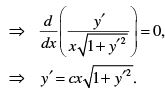Solving for y′ we get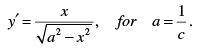Integrating we get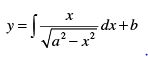Put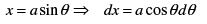. . . (5)

Therefore,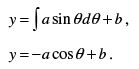. . . (6)

Squaring and adding equations (5) and (6) we get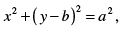which is the circle having center on y –axis.

Example 9 : Show that the geodesic on a right circular cylinder is a helix. Solution: We know the right circular cylinder is characterized by the equations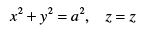. . . (1)

The parametric equations of the right circular cylinder are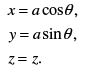where a is a constant. The element of the distance (metric)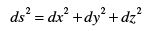on the surface of the cylinder becomes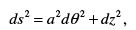Hence the total length of the curve on the surface of the cylinder is given by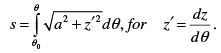. . . (2)
For s to be extremum, the integrand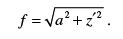. . . (3)

must satisfy the Euler-Lagrange’s equation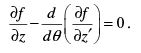. . . (4)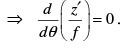Integrating the equation and solving for z′ we get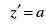(constant).
Integrating we get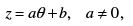. . . (5)

where a, b are constants. Equation (5) gives the required equation of helix. Thus the geodesic on the surface of a cylinder is a helix.

Example 10 : Find the differential equation of the geodesic on the surface of an inverted cone with semi-vertical angle θ .

Solution: The surface of the cone is characterized by the equation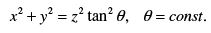. . . (1)

The parametric equations of the cone are given by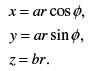. . . (2)

where for a = sinθ , b = cosθ are constant. Thus the metric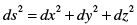on the surface of the cone becomes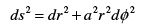. . . (3)

Hence the total length of the curve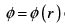on the surface of the cone is given by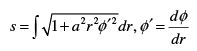. . . (4)

The length s is stationary if the integrand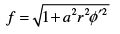. . . (5)

must satisfy the Euler-Lagrange’s equation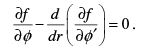. . . (6)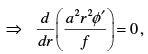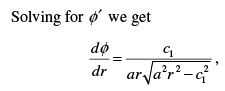. . . (7)

where  c1 = constant. This is the required differential equation of geodesic, and the geodesic on the surface of the cone is obtained by integrating equation (7). This gives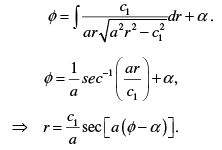Example 11 : Find the curve for which the functional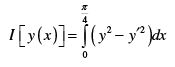can have extrema, given that y(0)=0, while the right –hand end point can vary along
the line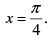Solution:  To find the extremal curve of the functional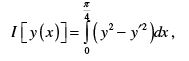. . . (1)
we must solve Euler’s equation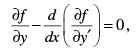. . . (2)
where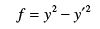. . . (3). . . (4)

This is the second order differential equation, whose solution is given by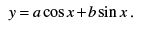. . . (5)

The boundary condition y ( 0 ) = 0 gives a = 0.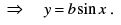. . . (6)
The second boundary point moves along the line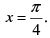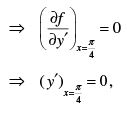where from equation (6) we have y′ = b cos x . Thusgives

b= 0. This implies the extremal is attained on the line y = 0.

Example 12 : If f satisfies Euler-Lagrange’s equation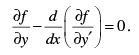Then show that f is the total derivative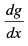of some function of x and y and conversely.

Solution:  Given that f satisfies Euler-Lagrange’s equation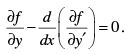. . . (1)

We claim that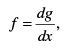where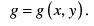As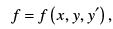we write equation (1) explicitly as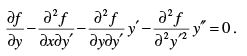. . . (2)
We see from equation (2) that the first three terms on the l. h. s. of (2) contain at the highest the first derivative of y. Therefore equation (2) is satisfied identically if the coefficient of y′′ vanishes identically.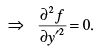Integrating w. r. t. y′ we get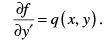Integrating once again we get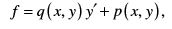. . (3)

where p ( x, y ) and q ( x, y ) are constants of integration and may be function of x and y only. Then the function f so determined must satisfy the Euler –Lagrange’s equation (1). From equation (3) we find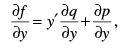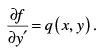and

Therefore equation (1) becomes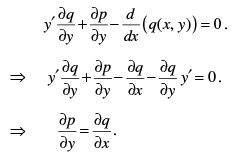.(4)

This is the condition that the equation pdx + qdy is an exact differential
equation dg .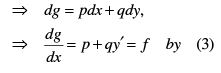Therefore,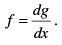. . . (5)
This proves the necessary part.

Conversely, assume that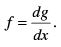We prove that f satisfies the Euler-Lagrange’s equation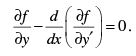Since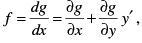Therefore, we find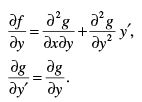Consider now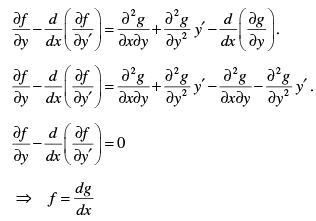satisfies Euler-Lagrange’s equation.

Example 13 : Show that the Euler-Lagrange’s equation of the functional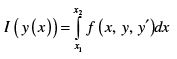has the first integral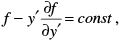if the integrand does not depend on x.

Solution: The Euler-Lagrange’s equation of the functional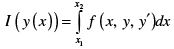to be extremum is given by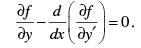. . . (1)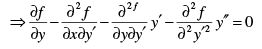If f does not involve x explicitly, then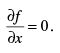Therefore, we have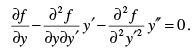. . . (2)

Multiply equation (2) by y′ we get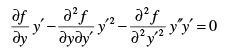. . . (3)
But we know that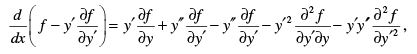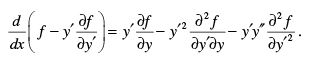. . . (4)

From equations (3) and (4) we see that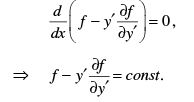. . . (5)
This is the first integral of Euler-Lagrange’s equation, when the functional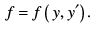Example 14 : Find the minimum of the functional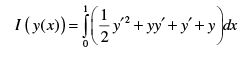if the values at the end points are not given.

Solution: For the minimum of the functional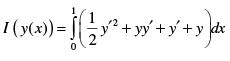. . . (1)
the integrand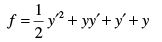. . . (2)
must satisfy the Euler-Lagrange’s equation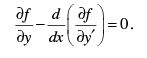. . . (3)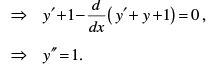. . . (4)
Integrating we get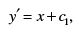. . . (5)
Further integrating we get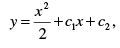. . . (6)

where c, c2 are constants of integration and are to be determined.

However, note that the values of y at the end points are not prescribed. In this case the constants are determined from the conditions.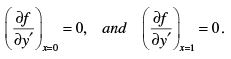. . . (7)
These two conditions will determine the values of the constants.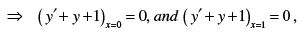. . . (8)
where from equation (5) and (6) we have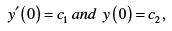similarly,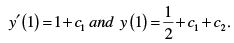Thus the equations (8) become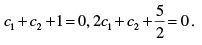Solving these equations for cand c2 we obtain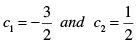Hence the required curve for which the functional given in (1) becomes minimum is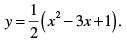. . . (9)

159 docs

,

,

,

,

,

,

,

,

,

,

,

,

,

,

,

,

,

,

,

,

,

,

,

,

;